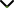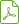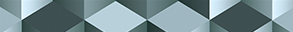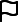# Recent Developments on Fixed Point Theory in Function Spaces and Applications to Control and Optimization Problems

Status
Published

Guest Editors

1Disha Institute of Management and Technology, Raipur, India

2University of Pretoria, Pretoria, South Africa

4Università degli Studi di Palermo, Palermo, Italy

# Recent Developments on Fixed Point Theory in Function Spaces and Applications to Control and Optimization Problems

## DescriptionNonlinear and Convex Analysis have as one of their goals solving equilibrium problems arising in various sciences, such as economics, physics, chemistry, and many others. A lot of these problems can be modelled in an abstract form of an equation (algebraic, functional, differential, integral, integrodifferential, etc.), and this can be further transferred into a form of a fixed point problem of a certain operator. In this context, finding solutions of fixed point problems, or at least proving that such solutions exist and can be approximately computed, is a very interesting area of research.

Indeed, one often comes across many problems with an operator equation where the exact solutions can neither be determined explicitly nor can be computed conveniently. In such situation, the following query logically takes place: does there exist an exact solution to the equation? Or a deeper question: how many different admissible solutions the given equation has? After obtaining an affirmative answer to the problem of existence, one can try to determine the solution as the limit of an iteration procedure.

Thus, the fixed point theory is part of both pure and applied mathematics. In addition of being part of Classical Analysis, Functional Analysis, Operator Theory, and Topology and Variational Inequality Theory, it is also used in new areas of mathematical applications like Mathematical Economics, Game Theory, Fluid flow, Eigen value problems, Random Differential Equations, and so forth. For instance, most of the theorems ensuring the existence of solutions to differential equations, integral equations, or other operator equations can be proved by using fixed point methods in specific abstract and function spaces.

The aim of this special issue is to promote research and its applications in the area of nonlinear functional analysis and applications, in particular to control and optimization problems. It will reflect theoretical research and advanced applications.

Potential topics include, but are not limited to:

• Study of fixed points and fixed point spaces
• Metric fixed point theorems and applications
• Random partial differential equations and applications
• Operator equations and inclusion problems
• Best approximation theorems in abstract spaces
• Convergence and stability of iteration procedures
• Semilinear control problemsDownload Special IssueJournal metrics
Acceptance rate28%
Submission to final decision43 days
Acceptance to publication37 days
CiteScore2.000
Impact Factor1.896Author guidelinesEditorial boardDatabases and indexingSign up for content alerts

We are committed to sharing findings related to COVID-19 as quickly as possible. We will be providing unlimited waivers of publication charges for accepted research articles as well as case reports and case series related to COVID-19. Review articles are excluded from this waiver policy. Sign up here as a reviewer to help fast-track new submissions.